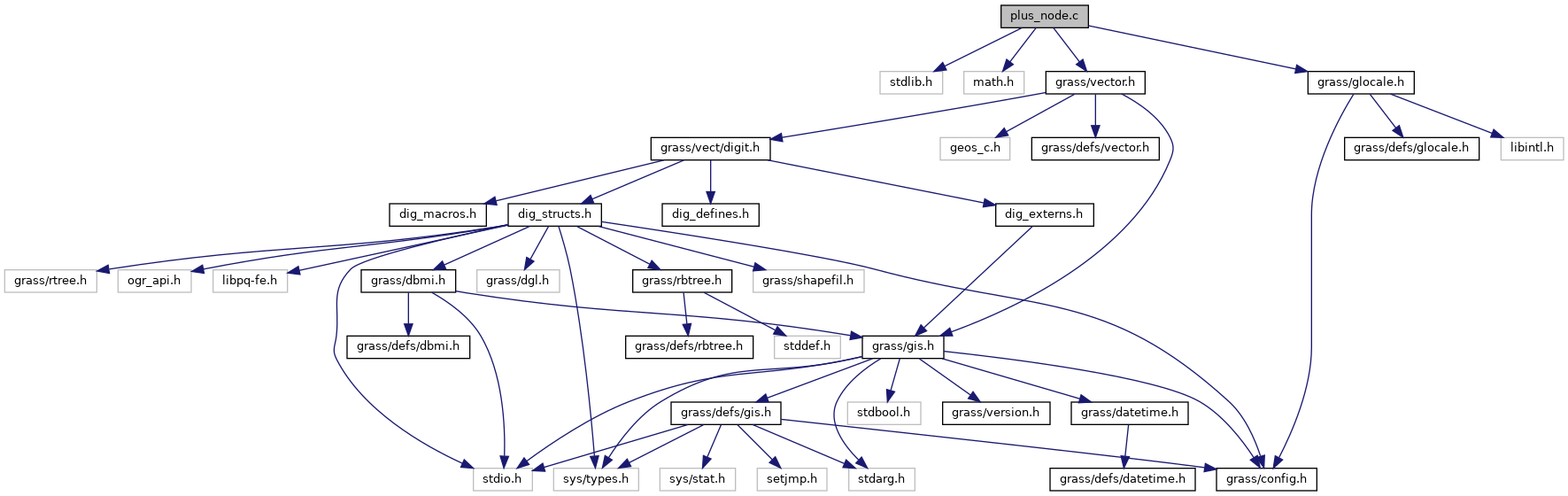GRASS GIS 7 Programmer's Manual  7.9.dev(2021)-e5379bbd7
plus_node.c File Reference

Vector library - update topo for nodes (lower level functions) More...

`#include <stdlib.h>`
`#include <math.h>`
`#include <grass/vector.h>`
`#include <grass/glocale.h>`
Include dependency graph for plus_node.c:Go to the source code of this file.

## Functions

int dig_node_add_line (struct Plus_head *plus, int nodeid, int lineid, const struct line_pnts *points, int type)
Add line info to node. More...

int dig_add_node (struct Plus_head *plus, double x, double y, double z)
Add new node to plus structure. More...

int dig_which_node (struct Plus_head *plus, double x, double y, double thresh)
Return actual index into node arrays of the first set of matching coordinates. More...

float dig_node_line_angle (struct Plus_head *plus, int nodeid, int lineid)
Return line angle. More...

## Detailed Description

Vector library - update topo for nodes (lower level functions)

Lower level functions for reading/writing/manipulating vectors.

This program is free software under the GNU General Public License (>=v2). Read the file COPYING that comes with GRASS for details.

Date
2001-2006

Definition in file plus_node.c.

## ◆ dig_add_node()

 int dig_add_node ( struct Plus_head * plus, double x, double y, double z )

Add new node to plus structure.

Parameters
 [in] plus pointer to Plus_head structure [in] x,y,z coordinates
Returns
-1 on error
number of node

Definition at line 102 of file plus_node.c.

## ◆ dig_node_add_line()

 int dig_node_add_line ( struct Plus_head * plus, int nodeid, int lineid, const struct line_pnts * points, int type )

Add line info to node.

Line will be negative if END node

'node' must of course already exist space will be alloced to add 'line' to array

Lines are sorted in increasing angle order and degenerated lines are set to -9 (ignored).

Parameters
 [in] plus pointer to Plus_head structure [in] nodeid node id [in] lineid line id [in] points line geometry [in] type line type
Returns
-1 on error
0 line not added (degenerate)
new number of lines in node

Definition at line 45 of file plus_node.c.

## ◆ dig_node_line_angle()

 float dig_node_line_angle ( struct Plus_head * plus, int nodeid, int lineid )

Return line angle.

Lines is specified by line id in topology, NOT by order number. Negative id if looking for line end point.

Parameters
 [in] plus pointer to Plus_head structure [in] nodeid node id [in] lineid line id
Returns
line angle <-PI,PI>
0 not reached

Definition at line 198 of file plus_node.c.

References _, P_node::angles, G_debug(), G_fatal_error(), P_node::lines, P_node::n_lines, and Plus_head::Node.

## ◆ dig_which_node()

 int dig_which_node ( struct Plus_head * plus, double x, double y, double thresh )

Return actual index into node arrays of the first set of matching coordinates.

Parameters
 [in] plus pointer to Plus_head structure [in] x,y coordinates [in] thresh threshold value
Returns
node index
-1 if no node found

Definition at line 146 of file plus_node.c.

References Plus_head::n_nodes, Plus_head::Node, NULL, P_node::x, and P_node::y.